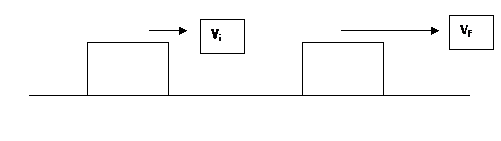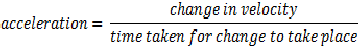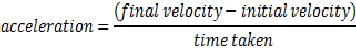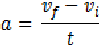Custom Search
If you want to discuss more on this or other issues related to Physics, feel free to leave a message on my Facebook page.

## Monday, February 1, 2010

### what is acceleration?

Acceleration is a term that is associated with a change with velocity of an object.

It is defined as the rate of change of velocity with time.

So it means that in order for an object to accelerate you need to have a change of velocity over a period of time.

There are three ways in which an object can accelerate that is its velocity can change over time.

1. Firstly as shown in fig 1 below the object’s velocity can increase with time.Fig 1

2. Secondly as shown in fig 2 below the velocity of the object can decrease with time.Fig 2

3. Lastly the velocity of the object can change due to a change in direction as shown below in fig 3. Remember the velocity is a vector quantity and as a result has a direction component and a magnitude component. Even if the magnitude remain constant a change in direction will result in a change in the velocity.

Fig 3

However we will only consider the first two cases today. The third one will be considered when we study circular motion.

If an object has a velocity v1 is subjected to a force such that after a period of time t of being subjected to the force its velocity becomes v2 then we can say that the object is experiencing an acceleration.

This acceleration can thus be calculated using the equationFrom this equation we can thus see that when the velocity of an object increases then the acceleration is a positive number and when the velocity of the object decreases the acceleration is a negative number.

The acceleration is a vector quantity since it is a vector quantity divide by a scalar quantity.